# Configuring a Transient Analysis in Multisim

Publish Date: Mar 27, 2012 | 7 Ratings | 4.00 out of 5 | Print | Submit your review

## Overview

Multisim features a comprehensive suite of SPICE analyses for examining circuit behavior. These analyses range from the basic to sophisticated. Each analysis helps you to obtain valuable information such as the effects of component tolerances and sensitivities. For each analysis you need to set settings that will inform Multisim exactly what to analyze, and how.

Multisim simplifies the procedure for an advanced analysis by providing a configuration window. This abstracts away the complexities associated with SPICE syntax and configuration of an analysis. With this window you merely need to specify the parameter values and output nodes of interest.

This tutorial is part of the National Instruments SPICE Analysis Fundamentals Series. Each tutorial in this series provides you with step-by-step instructions on how to configure and run the different SPICE analyses available in Multisim.powerful simulation and analysis while abstracting the complexity of SPICE syntax.

### 1. Introduction

In Transient Analysis, also called time-domain transient analysis, Multisim computes the circuit’s response as a function of time. This analysis divides the time into segments and calculates the voltage and current levels for each given interval. Finally, the results, voltage versus time, are presented in the Grapher View.

Multisim performs Transient Analysis using the following process:

1. Each input cycle is divided into intervals.
2. A DC Operating Point Analysis is performed for each time point in the cycle.
3. The solution for the voltage waveform at a node is determined by the value of that voltage at each time point over one complete cycle.

Assumptions: DC sources have constant values; AC sources have time-dependent values. Capacitors and inductors are represented by energy storage models. Numerical integration is used to calculate the quantity of energy transfer over an interval of time.

### 2. Running Transient Analysis

Consider the series RLC circuit shown in Figure 1. According to the theory, the characteristic equation modeling this circuit can be represented as: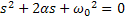Where α is the damping factor and w0  the natural frequency (or resonant frequency). They are defined by: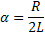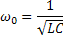The value of the damping factor (α) in relation to the natural frequency (ω0) determines the behavior of the circuit’s response. There are three possible responses:

• α < ω0 : Underdamped response
• α = ω0 : Critically damped response
• α > ω0 : Overdamped response

Note that as the value of α increases, the RLC circuit is driven towards an overdamped response. In this example you will use Transient Analysis to plot the step responses of the RLC circuit. Since α depends on the value of the resistance, you will use three different values for R: 40 W, 200 W and 1 kW.  3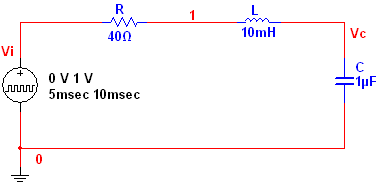Figure 1. Series RLC circuit.

Complete the following steps to configure and run a Transient Analysis:

2. Select Simulate»Analyses»Transient Analysis. The Transient Analysis window opens.

The default settings are appropriate for normal use, providing the transient response of the selected output variables starting at time 0 seconds and stopping after 1 ms. Table 1 describes the Analysis Parameters tab in detail.

Table 1. Parameters used in Transient Analysis.

 Parameter Meaning Initial Conditions There are four options: Set to zero. The analysis starts from zero initial conditions. User-defined. The analysis starts from initial conditions as set in the Transient Analysis window. Calculate DC operating point. Multisim first calculates the DC operating point of the circuit, then uses that result as the initial conditions of the Transient Analysis. Automatically determine initial conditions. Multisim tries to start the simulation using the DC operating point as the initial condition. If the simulation fails, it uses user-defined initial conditions. Start time (TSTART) Start time of Transient Analysis. Must be greater than or equal to 0 and less than end time. End time (TSTOP) End time of Transient Analysis. Must be greater than start time. Maximum time step settings (TMAX) Enable to manually set time steps.  There are three options: Minimum number of time points. Minimum number of points between start and stop times. Maximum time step (TMAX). Maximum time step the simulation can handle. Generate time steps automatically. Enable to generate time steps automatically. Set initial time step (TSTEP) Enable to set a time interval for simulation output and graphing. Estimate maximum time step based on netlist (TMAX) This becomes enabled when the Maximum timestep (TMAX) checkbox is selected. Enable as desired.

Note: In SPICE, the command that performs a Transient Analysis has the following form:

.TRAN <TSTEP> <TSTOP> < TSTART <TMAX> > <UIC>

Where .TRAN initializes a Transient Analysis; <TSTEP> is the time increment for reporting results; <TSTOP> is the final analysis time; <TSTART> is the start time for reporting results; <TMAX> is the maximum step size used in incrementing the time during the analysis; <UIC> is used for initial conditions. Note that these are the same parameters that were defined in Table 1, however, in Multisim you do not have to worry about the complex SPICE syntax.

1. Configure the Analysis Parameters as shown in Figure 2. You can reset all the parameters to their default values by clicking the Reset to default button.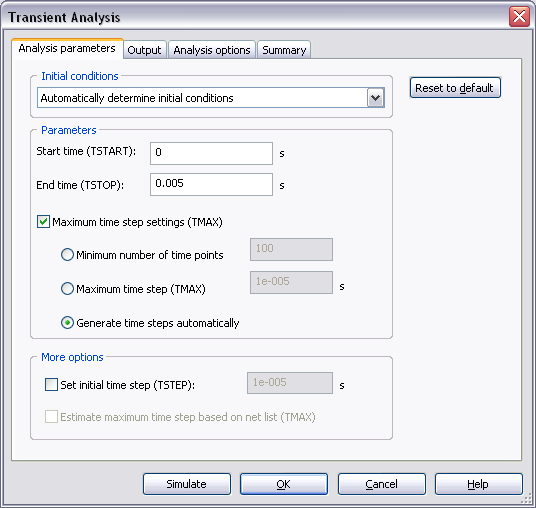Figure 2. Analysis parameters for the Transient Analysis.

1. Select the Output tab.
2. Select the Variables in circuit list, select All variables from the drop-down list, and then highlight V(vi) and V(c) from the list.
3. Click the Add button to move the variables to the right side under Selected variables for analysis, as shown below.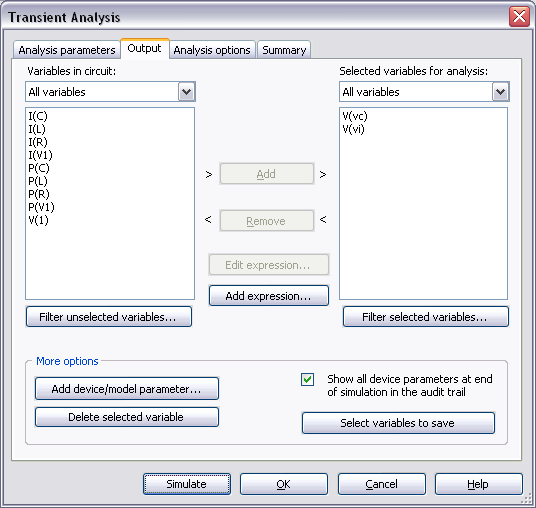Figure 3. Output variables for the Transient Analysis.

1. Click Simulate. The Grapher View window opens. Results are displayed in Figure 4.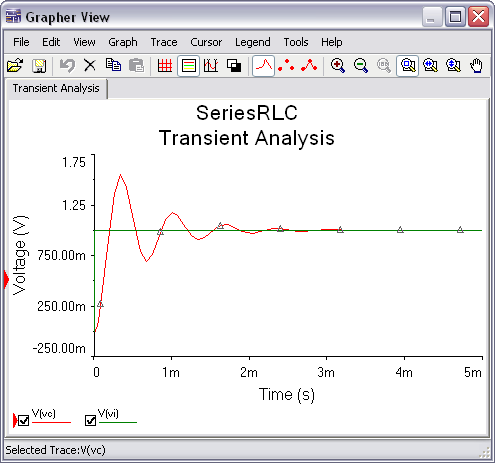Figure 4. Transient Analysis results.

As you can see, this is the typical underdamped response of a series RLC circuit.

Note: If you connect the Oscilloscope to the circuit and run the simulation, a similar analysis is performed.

1. Close the Grapher View.
2. Change the value of R to 200 W.
3. Run Transient Analysis once again. You will see the critically damped response.
4. Run Transient Analysis for R = 1 kW. The overdamped response will be plotted.

In order to compare the three results, merge the plots in one. You can use Overlay Traces from the Graph menu. Figure 5 shows a comparison graph of the results.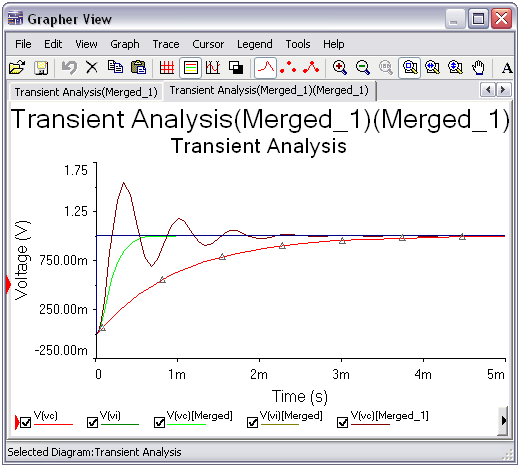Figure 5. Step responses of the RLC circuit.

In this example you executed the simulation three times in order to get the step responses of the RLC circuit, however, you can also use Parameter Sweep Analysis to verify the behavior of a circuit when a parameter is varied across a range of values.

Entering Expressions in Analyses in Multisim

SPICE Analysis Fundamentals

Circuit Design Technical Library

Join the NI Circuit Design Community